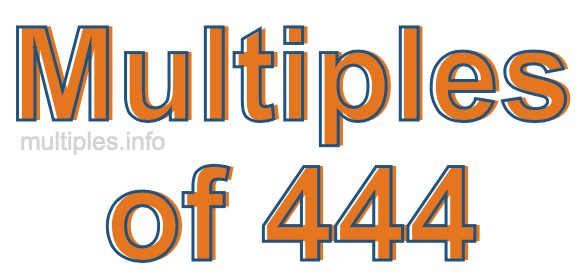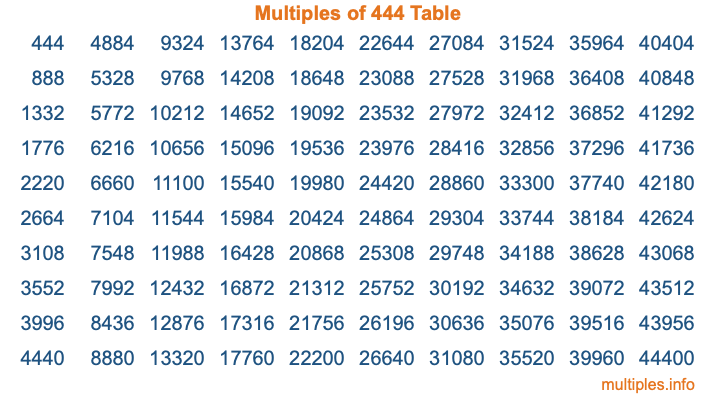Multiples of 444Welcome to the Multiples of 444 page. Here we will first teach you everything you will ever need to know about the multiples of 444, and then give you a study guide summary of everything we taught you to make sure you remember it all. Use this page to look up facts and learn information about the multiples of 444. This page will make you a multiples of four hundred forty-four expert!

Definition of Multiples of 444
Multiples of 444 are all the numbers that when divided by 444 equal an integer. Each of the multiples of 444 are called a multiple. A multiple of 444 is created by multiplying 444 by an integer.

Therefore, to create a list of multiples of 444, you start with 1 multiplied by 444, then 2 multiplied by 444, then 3 multiplied by 444, and so on for as long as you want. Thus, the list of the first five multiples of 444 is 444, 888, 1332, 1776, and 2220. To see a larger list of multiples of 444, see the printable image of Multiples of 444 further down on this page. We also have a category where you can choose any nth multiple of 444.

Multiples of 444 Checker
The Multiples of 444 Checker below checks to see if any number of your choice is a multiple of 444. In other words, it checks to see if there is any number (integer) that when multiplied by 444 will equal your number. To do that, we divide your number by 444. If the the quotient is an integer, then your number is a multiple of 444.

Is  a multiple of 444?

Least Common Multiple of 444 and ...
A Least Common Multiple (LCM) is the lowest multiple that two or more numbers have in common. This is also called the smallest common multiple or lowest common multiple and is useful to know when you are adding our subtracting fractions. Enter one or more numbers below (444 is already entered) to find the LCM.

Check out our LCM Calculator if you need more details about the Least Common Multiple or if you need the LCM for different numbers for adding and subtraction fractions.

nth Multiple of 444
As we stated above, 444 is the first multiple of 444, 888 is the second multiple of 444, 1332 is the third multiple of 444, and so on. Enter a number below to find the nth multiple of 444.

th multiple of 444

Multiples of 444 vs Factors of 444
444 is a multiple of 444 and a factor of 444, but that is where the similarities end. All postive multiples of 444 are 444 or greater than 444. All positive factors of 444 are 444 or less than 444.

Below is the beginning list of multiples of 444 and the factors of 444 so you can compare:

Multiples of 444: 444, 888, 1332, 1776, 2220, etc.

Factors of 444: 1, 2, 3, 4, 6, 12, 37, 74, 111, 148, 222, 444

As you can see, the multiples of 444 are all the numbers that you can divide by 444 to get a whole number. The factors of 444, on the other hand, are all the whole numbers that you can multiply by another whole number to get 444.

It's also interesting to note that if a number (x) is a factor of 444, then 444 will also be a multiple of that number (x).

Multiples of 444 vs Divisors of 444
The divisors of 444 are all the integers that 444 can be divided by evenly. Below is a list of the divisors of 444.

Divisors of 444: 1, 2, 3, 4, 6, 12, 37, 74, 111, 148, 222, 444

The interesting thing to note here is that if you take any multiple of 444 and divide it by a divisor of 444, you will see that the quotient is an integer.

Multiples of 444 Table
Below is an image of the first 100 multiples of 444 in a table. The table is in chronological order, column by column. The first column has the first ten multiples of 444, the second column has the next ten multiples of 444, and so on.The Multiples of 444 Table is also referred to as the 444 Times Table or Times Table of 444. You are welcome to print out our table for your studies.

Negative Multiples of 444
Although not often discussed or needed in math, it is worth mentioning that you can make a list of negative multiples of 444 by multiplying 444 by -1, then by -2, then by -3, and so on, to get the following list of negative multiples of 444:

-444, -888, -1332, -1776, -2220, etc.

Multiples of 444 Summary
Below is a summary of important Multiples of 444 facts that we have discussed on this page. To retain the knowledge on this page, we recommend that you read through the summary and explain to yourself or a study partner why they hold true.

There are an infinite number of multiples of 444.

A multiple of 444 divided by 444 will equal a whole number.

444 divided by a factor of 444 equals a divisor of 444.

The nth multiple of 444 is n times 444.

The largest factor of 444 is equal to the first positive multiple of 444.

444 is a multiple of every factor of 444.

444 is a multiple of 444.

A multiple of 444 divided by a divisor of 444 equals an integer.

444 divided by a divisor of 444 equals a factor of 444.

Any integer times 444 will equal a multiple of 444.

Multiples of a Number
Here you can get the multiples of another number, all with the same attention to detail as we did for multiples of 444 on this page.

Multiples of
Multiples of 445
Did you find our page about multiples of four hundred forty-four educational? Do you want more knowledge? Check out the multiples of the next number on our list!